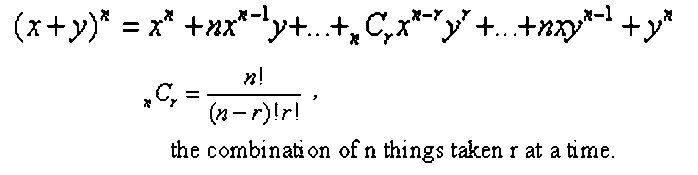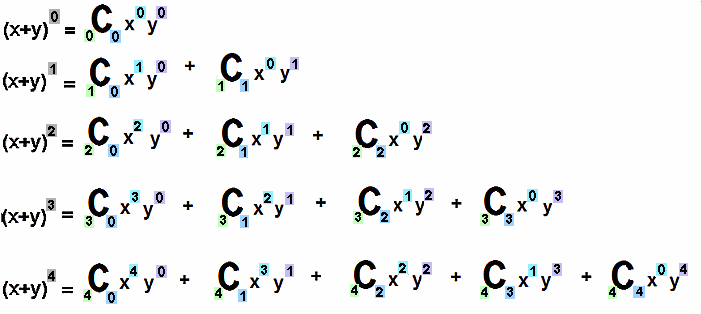The Visual / Auditory / Symbolic / Kinesthetic Approach to Algebra

 Binomial Expansion Presented Symbolically and Pascal's Triangle

Extensive nonTile work has laid the foundation for this and the next pages. Combinations and different sets of numbers abound in the expansion of a binomial and Pascal's Triangle. Think of it as the "mile deep" background a teacher should have before the "inch or more wide" presentation of the binomial expansion.

Because the next page presents warm-up, lesson, and summary, and extension on binomial expansion, this page serves only as a reference once the next lesson has begun. Think of this as the resource that will be used for the summary and extension of the next page. Without additional comment, the Binomial Expansion Presented Symbolically and Pictorially.

The sum of two terms, x + y, raised to the 0, 1st, 2nd, 3rd, 4th, and 5th powers is stated below.Generate the nth row of binomial expansion (the sum of two terms multiplied a certain number of times and written in expanded form) using:Restated with more color and detail.The sum of two tems, x + y, raised to the 0, 1st, 2nd, 3rd, 4th, and 5th powers is stated below.Pascal's Triangle

Pascal's Triangle provides the coefficients of the terms in the expansion of a binomial.The coefficient of the terms are the sum of the pair of terms surrounding the term, but, in the row above it.Many different sets of numbers are found in Pascal's Triangle. These include the natural numbers, triangle numbers, and the sum of the triangle numbers. See the page on "Sets of Numbers" for more info.www.termtiles.com, Unit 43   © 2008, A. Azzolino